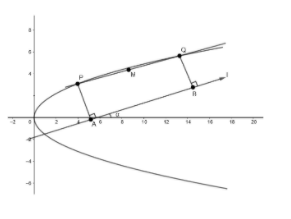Courses
Courses for Kids
Free study material
Free LIVE classes
More# Prove that the locus of the poles of chords which subtend a right angle at a fixed point $\left( h,k \right)$ is $a{{x}^{2}}-h{{y}^{2}}+\left( 4{{a}^{2}}+2ah \right)x-2aky+a\left( {{h}^{2}}+{{k}^{2}} \right)=0$

Last updated date: 24th Mar 2023
Total views: 308.1k
Views today: 6.86kVerified
308.1k+ views
Hint: Length of projection of $\overrightarrow{a}$ on $\overrightarrow{b}$is given as $\left| \dfrac{\overrightarrow{a}.\overrightarrow{b}}{\left| \overrightarrow{b} \right|} \right|$.

We will consider the equation of the parabola to be ${{y}^{2}}=4ax$.
We know , the equation of axis of this parabola is $y=0$Let us assume two points $P\left( at_{1}^{2},2a{{t}_{1}} \right)$ and $Q\left( at_{2}^{2},2a{{t}_{2}} \right)$ on the parabola.
Now, we will find the equation of chord $PQ$ in vector form.
We know , the equation of line joining the points $({{x}_{1}},{{y}_{1}})$ and $({{x}_{2}},{{y}_{2}})$, in vector form , is given as
$\overrightarrow{L}=({{x}_{2}}-{{x}_{1}})\widehat{i}+({{y}_{2}}-{{y}_{1}})\widehat{j}$.
So , the equation of the line joining $P\left( at_{1}^{2},2a{{t}_{1}} \right)$ and $Q\left( at_{2}^{2},2a{{t}_{2}} \right)$ is given as
$\overrightarrow{PQ}=\left( at_{2}^{2}-at_{1}^{2} \right)\hat{i}+\left( 2a{{t}_{2}}-2a{{t}_{1}} \right)\hat{j}$
Now, we need to find the locus of the midpoint of $PQ$.
So , let the midpoint of $PQ$ be $M\left( h,k \right)$.
Now, we know that the coordinates of the midpoint of the line joining two points $\left( {{x}_{1}},{{y}_{1}} \right)$ and $\left( {{x}_{2}},{{y}_{2}} \right)$ is given as: $\left( \dfrac{{{x}_{1}}+{{x}_{2}}}{2},\dfrac{{{y}_{1}}+{{y}_{2}}}{2} \right)$
So , $h=\dfrac{at_{1}^{2}+at_{2}^{2}}{2}....\left( i \right)$ and $k=\dfrac{2a{{t}_{1}}+2a{{t}_{2}}}{2}....\left( ii \right)$
From $\left( i \right)$ we get $\left( at_{1}^{2}+at_{2}^{2} \right)=2h$
From $\left( ii \right)$ we get $\left( a{{t}_{1}}+a{{t}_{2}} \right)=k$
Now , we will find the projection of $\overrightarrow{PQ}$ on line $l$.
Let this projection be $\overrightarrow{AB}$.
Now , in the question, it is given that the length of projection of chord on the line is a constant $C$.
We know , the length of projection of $\overrightarrow{a}$ on $\overrightarrow{b}$is given as
$\left| \dfrac{\overrightarrow{a}.\overrightarrow{b}}{\left| \overrightarrow{a} \right|} \right|$
So , the length of projection of $\overrightarrow{PQ}$ on $\overrightarrow{AB}$ is given as
$\left| \dfrac{\overrightarrow{PQ}.\overrightarrow{AB}}{\left| \overrightarrow{AB} \right|} \right|=C.....\left( iii \right)$
Now, we know the length of $\overrightarrow{AB}=C$ and line $AB$is inclined at angle $\alpha$to the axis.
We know , the equation of line of length $r$ and inclined at an angle $\theta$ with the $x$-axis is given as
$\overrightarrow{L}=r\cos \theta \widehat{i}+r\sin \theta \widehat{j}$
So , equation of $\overrightarrow{AB}$in vector form is
$\overrightarrow{AB}=C\cos \alpha \hat{i}+C\sin \alpha \hat{j}$
Substituting the equation of $\overrightarrow{AB}$ in equation $\left( iii \right)$, we get
$\left| \dfrac{\left( \left( at_{2}^{2}-at_{1}^{2} \right)\hat{i}+\left( 2a{{t}_{2}}-2a{{t}_{1}} \right)\hat{j} \right).\left( C\cos \alpha \hat{i}+C\sin \alpha \hat{j} \right)}{C} \right|=C$
$\Rightarrow \left| \dfrac{C\left( at_{2}^{2}-at_{1}^{2} \right)\cos \alpha +C\sin \alpha \left( 2a{{t}_{2}}-2a{{t}_{2}} \right)}{C} \right|=C$
$\Rightarrow \left| a\left[ \left( t_{2}^{2}-t_{1}^{2} \right)\cos \alpha +2\sin \alpha \left( {{t}_{2}}-{{t}_{1}} \right) \right] \right|=C$
Now , we will square both sides to remove the modulus sign.
On squaring both sides, we get
${{a}^{2}}{{\left( {{t}_{2}}-{{t}_{1}} \right)}^{2}}{{\left[ \left( {{t}_{1}}+{{t}_{2}} \right)\cos \alpha +2\sin \alpha \right]}^{2}}={{C}^{2}}$
$\Rightarrow {{a}^{2}}\left( t_{2}^{2}+t_{2}^{2}-2{{t}_{1}}{{t}_{2}} \right)\left[ {{\left( {{t}_{1}}+{{t}_{2}} \right)}^{2}}{{\cos }^{2}}\alpha +4{{\sin }^{2}}\alpha +4\left( {{t}_{1}}+{{t}_{2}} \right)\cos \alpha \sin \alpha \right]={{C}^{2}}.....(iv)$
Now , we know $a\left( t_{1}^{2}+t_{2}^{2} \right)=2h$ [from $(i)$]
$\Rightarrow t_{1}^{2}+t_{2}^{2}=\dfrac{2h}{a}$
And $a\left( {{t}_{1}}+{{t}_{2}} \right)=k$[from $(ii)$]
$\Rightarrow {{t}_{1}}+{{t}_{2}}=\dfrac{k}{a}$
$\Rightarrow t_{1}^{2}+t_{2}^{2}+2{{t}_{1}}{{t}_{2}}=\dfrac{{{k}^{2}}}{{{a}^{2}}}$
$\Rightarrow 2{{t}_{1}}{{t}_{2}}=\dfrac{{{k}^{2}}}{{{a}^{2}}}-\dfrac{2h}{a}$
Substituting in $\left( iv \right)$, we get
${{a}^{2}}\left( \dfrac{2h}{a}-\dfrac{{{k}^{2}}}{{{a}^{2}}}+\dfrac{2h}{a} \right)\left[ {{\left( \dfrac{k}{a} \right)}^{2}}{{\cos }^{2}}\alpha +4{{\sin }^{2}}\alpha +4\dfrac{k}{a}\cos \alpha \sin \alpha \right]={{C}^{2}}$
$\Rightarrow \left( 4ah-{{k}^{2}} \right)\left[ {{k}^{2}}{{\cos }^{2}}\alpha +4{{a}^{2}}{{\sin }^{2}}\alpha +4ak\cos \alpha \sin \alpha \right]={{a}^{2}}{{C}^{2}}$
$\Rightarrow \left( 4ah-{{k}^{2}} \right){{\left[ k\cos \alpha +2a\sin \alpha \right]}^{2}}={{a}^{2}}{{C}^{2}}.........$ equation$(v)$
Now , to get the equation of the locus of $M\left( h,k \right)$, we will substitute $(x,y)$in place of $\left( h,k \right)$ in equation $(v)$.
So , the locus of $M\left( h,k \right)$ is given as
$\left( 4ax-{{y}^{2}} \right){{\left( y\cos \alpha +2a\sin \alpha \right)}^{2}}={{a}^{2}}{{C}^{2}}$
Or $\left( {{y}^{2}}-4ax \right){{\left( y\cos \alpha +2a\sin \alpha \right)}^{2}}+{{a}^{2}}{{C}^{2}}=0$
Note: While simplifying the equations , please make sure that sign mistakes do not occur. These mistakes are very common and can cause confusions while solving. Ultimately the answer becomes wrong. So, sign conventions should be carefully taken